Search by Topic

Resources tagged with Interactivities similar to Cubic Net:

Filter by: Content type:
Age range:
Challenge level:

There are 106 results

Broad Topics > Information and Communications Technology > InteractivitiesCubic Net

Age 14 to 18 Challenge Level:

This is an interactive net of a Rubik's cube. Twists of the 3D cube become mixes of the squares on the 2D net. Have a play and see how many scrambles you can undo!Inside Out

Age 14 to 16 Challenge Level:

There are 27 small cubes in a 3 x 3 x 3 cube, 54 faces being visible at any one time. Is it possible to reorganise these cubes so that by dipping the large cube into a pot of paint three times you. . . .Changing Places

Age 14 to 16 Challenge Level:

Place a red counter in the top left corner of a 4x4 array, which is covered by 14 other smaller counters, leaving a gap in the bottom right hand corner (HOME). What is the smallest number of moves. . . .Square It

Age 11 to 16 Challenge Level:

Players take it in turns to choose a dot on the grid. The winner is the first to have four dots that can be joined to form a square.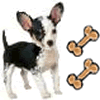Ratio Pairs 3

Age 11 to 16 Challenge Level:

Match pairs of cards so that they have equivalent ratios.Jam

Age 14 to 16 Challenge Level:

To avoid losing think of another very well known game where the patterns of play are similar.Sliding Puzzle

Age 11 to 16 Challenge Level:

The aim of the game is to slide the green square from the top right hand corner to the bottom left hand corner in the least number of moves.3D Treasure Hunt

Age 14 to 18 Challenge Level:

Some treasure has been hidden in a three-dimensional grid! Can you work out a strategy to find it as efficiently as possible?Instant Insanity

Age 11 to 18 Challenge Level:

Given the nets of 4 cubes with the faces coloured in 4 colours, build a tower so that on each vertical wall no colour is repeated, that is all 4 colours appear.Just Rolling Round

Age 14 to 16 Challenge Level:

P is a point on the circumference of a circle radius r which rolls, without slipping, inside a circle of radius 2r. What is the locus of P?White Box

Age 7 to 18 Challenge Level:

This game challenges you to locate hidden triangles in The White Box by firing rays and observing where the rays exit the Box.Picturing Triangular Numbers

Age 11 to 14 Challenge Level:

Triangular numbers can be represented by a triangular array of squares. What do you notice about the sum of identical triangle numbers?Icosian Game

Age 11 to 14 Challenge Level:

This problem is about investigating whether it is possible to start at one vertex of a platonic solid and visit every other vertex once only returning to the vertex you started at.Fractions and Percentages Card Game

Age 11 to 16 Challenge Level:

Match the cards of the same value.Colour in the Square

Age 7 to 16 Challenge Level:

Can you put the 25 coloured tiles into the 5 x 5 square so that no column, no row and no diagonal line have tiles of the same colour in them?Cogs

Age 11 to 14 Challenge Level:

A and B are two interlocking cogwheels having p teeth and q teeth respectively. One tooth on B is painted red. Find the values of p and q for which the red tooth on B contacts every gap on the. . . .Tilting Triangles

Age 14 to 16 Challenge Level:

A right-angled isosceles triangle is rotated about the centre point of a square. What can you say about the area of the part of the square covered by the triangle as it rotates?Olympic Magic

Age 14 to 16 Challenge Level:

in how many ways can you place the numbers 1, 2, 3 … 9 in the nine regions of the Olympic Emblem (5 overlapping circles) so that the amount in each ring is the same?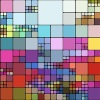Squaring the Rectangle

Age 14 to 18 Challenge Level:

Can you find a way to turn a rectangle into a square?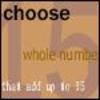Fifteen

Age 7 to 14 Challenge Level:

Can you spot the similarities between this game and other games you know? The aim is to choose 3 numbers that total 15.Excel Interactive Resource: Interactive Division

Age 11 to 16 Challenge Level:

Use an Excel to investigate division. Explore the relationships between the process elements using an interactive spreadsheet.Spinners Environment

Age 5 to 18 Challenge Level:

A tool for generating random integers.Countdown Fractions

Age 11 to 16 Challenge Level:

Here is a chance to play a fractions version of the classic Countdown Game.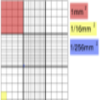Using the Haemocytometer

Age 14 to 16 Challenge Level:

Practise your skills of proportional reasoning with this interactive haemocytometer.Drips

Age 7 to 14 Challenge Level:

An animation that helps you understand the game of Nim.Konigsberg Plus

Age 11 to 14 Challenge Level:

Euler discussed whether or not it was possible to stroll around Koenigsberg crossing each of its seven bridges exactly once. Experiment with different numbers of islands and bridges.Number Pyramids

Age 11 to 14 Challenge Level:

Try entering different sets of numbers in the number pyramids. How does the total at the top change?Got a Strategy for Last Biscuit?

Age 11 to 16 Challenge Level:

Can you beat the computer in the challenging strategy game?Cushion Ball Interactivity

Age 14 to 16 Challenge Level:

The interactive diagram has two labelled points, A and B. It is designed to be used with the problem "Cushion Ball"Excel Interactive Resource: Fraction Multiplication

Age 11 to 16 Challenge Level:

Use Excel to explore multiplication of fractions.Loci Resources

Age 14 to 16 Challenge Level:

This set of resources for teachers offers interactive environments to support work on loci at Key Stage 4.Solving with Euclid's Algorithm

Age 14 to 18 Challenge Level:

A java applet that takes you through the steps needed to solve a Diophantine equation of the form Px+Qy=1 using Euclid's algorithm.Excel Interactive Resource: Long Multiplication

Age 11 to 16 Challenge Level:

Use an Excel spreadsheet to explore long multiplication.Excel Interactive Resource: Equivalent Fraction Bars

Age 11 to 16 Challenge Level:

A simple file for the Interactive whiteboard or PC screen, demonstrating equivalent fractions.Excel Interactive Resource: the up and Down Game

Age 11 to 16 Challenge Level:

Use an interactive Excel spreadsheet to explore number in this exciting game!Excel Interactive Resource: Number Grid Functions

Age 11 to 16 Challenge Level:

Use Excel to investigate the effect of translations around a number grid.Percentages for Key Stage 4

Age 14 to 16 Challenge Level:

A group of interactive resources to support work on percentages Key Stage 4.More Magic Potting Sheds

Age 11 to 14 Challenge Level:

The number of plants in Mr McGregor's magic potting shed increases overnight. He'd like to put the same number of plants in each of his gardens, planting one garden each day. How can he do it?Excel Interactive Resource: Fraction Addition & Fraction Subtraction

Age 11 to 16 Challenge Level:

Use Excel to practise adding and subtracting fractions.Factors and Multiples - Secondary Resources

Age 11 to 16 Challenge Level:

A collection of resources to support work on Factors and Multiples at Secondary level.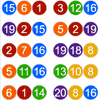Lottery Simulator

Age 7 to 16 Challenge Level:

Use this animation to experiment with lotteries. Choose how many balls to match, how many are in the carousel, and how many draws to make at once.Excel Interactive Resource: Haitch

Age 11 to 16 Challenge Level:

An Excel spreadsheet with an investigation.Excel Interactive Resource: Multiples Chain

Age 11 to 16 Challenge Level:

Use an interactive Excel spreadsheet to investigate factors and multiples.Semi-regular Tessellations

Age 11 to 16 Challenge Level:

Semi-regular tessellations combine two or more different regular polygons to fill the plane. Can you find all the semi-regular tessellations?Red or Black Spinner

Age 3 to 16 Challenge Level:

A simple spinner that is equally likely to land on Red or Black. Useful if tossing a coin, dropping it, and rummaging about on the floor have lost their appeal. Needs a modern browser; if IE then at. . . .Have You Got It?

Age 11 to 14 Challenge Level:

Can you explain the strategy for winning this game with any target?Countdown

Age 7 to 14 Challenge Level:

Here is a chance to play a version of the classic Countdown Game.Rollin' Rollin' Rollin'

Age 11 to 14 Challenge Level:

Two circles of equal radius touch at P. One circle is fixed whilst the other moves, rolling without slipping, all the way round. How many times does the moving coin revolve before returning to P?First Connect Three

Age 7 to 14 Challenge Level:

Add or subtract the two numbers on the spinners and try to complete a row of three. Are there some numbers that are good to aim for?Partitioning Revisited

Age 11 to 14 Challenge Level:

We can show that (x + 1)² = x² + 2x + 1 by considering the area of an (x + 1) by (x + 1) square. Show in a similar way that (x + 2)² = x² + 4x + 4